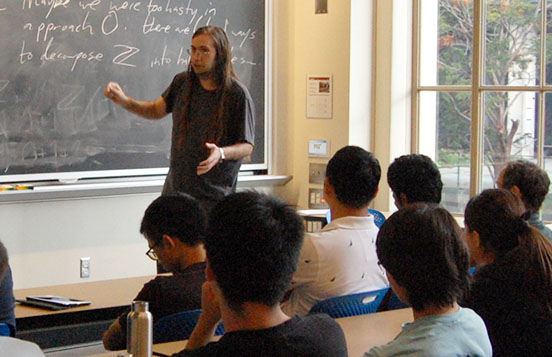# RSI/SPUR Lecture SeriesAaron Pixton's talk in the 2018 RSI/SPUR Lecture Series

This lecture series is organized by SPUR/RSI faculty advisors Davesh Maulik and Ankur Moitra. These summer lectures are aimed at our RSI and SPUR students.

• July 7, Larry Guth

Focusing waves and combinatorics of lines

Solutions of the wave equation model sound waves, light waves, etc. Focusing refers to the amplitude getting very large in a small region of space. Estimating how much waves can focus is a problem in real analysis. Over the last 25 years, mathematicians have approached this problem using ideas from combinatorics about the intersection patterns of lines. The story involves parts of math that sound rather far from PDE, such as some topology and some finite fields. In this talk, we will begin with a gentle introduction to waves and the wave equation, and then describe how ideas from some of these other fields come into play.

• July 21, Alexei Borodin

Domino tilings of the Aztec diamond

The talk is a survey of one of the most beautiful solvable probabilistic models and its relations with other fields - interacting particle systems, random interfaces in 3d, and random matrices.

• August 4, Lisa Sauermann

On the cap-set problem and the slice rank polynomial method

In 2016, Ellenberg and Gijswijt made a breakthrough on the famous cap-set problem, which asks about the maximum size of a subset of $\mathbb{F}_3^n$ not containing a three-term arithmetic progression. They proved that any such set has size at most $2.756^n$. Their proof was later reformulated by Tao, introducing what is now called the slice rank polynomial method. This talk will explain Tao's proof of the Ellenberg-Gijswijt bound for the cap-set problem, and discuss some related problems.